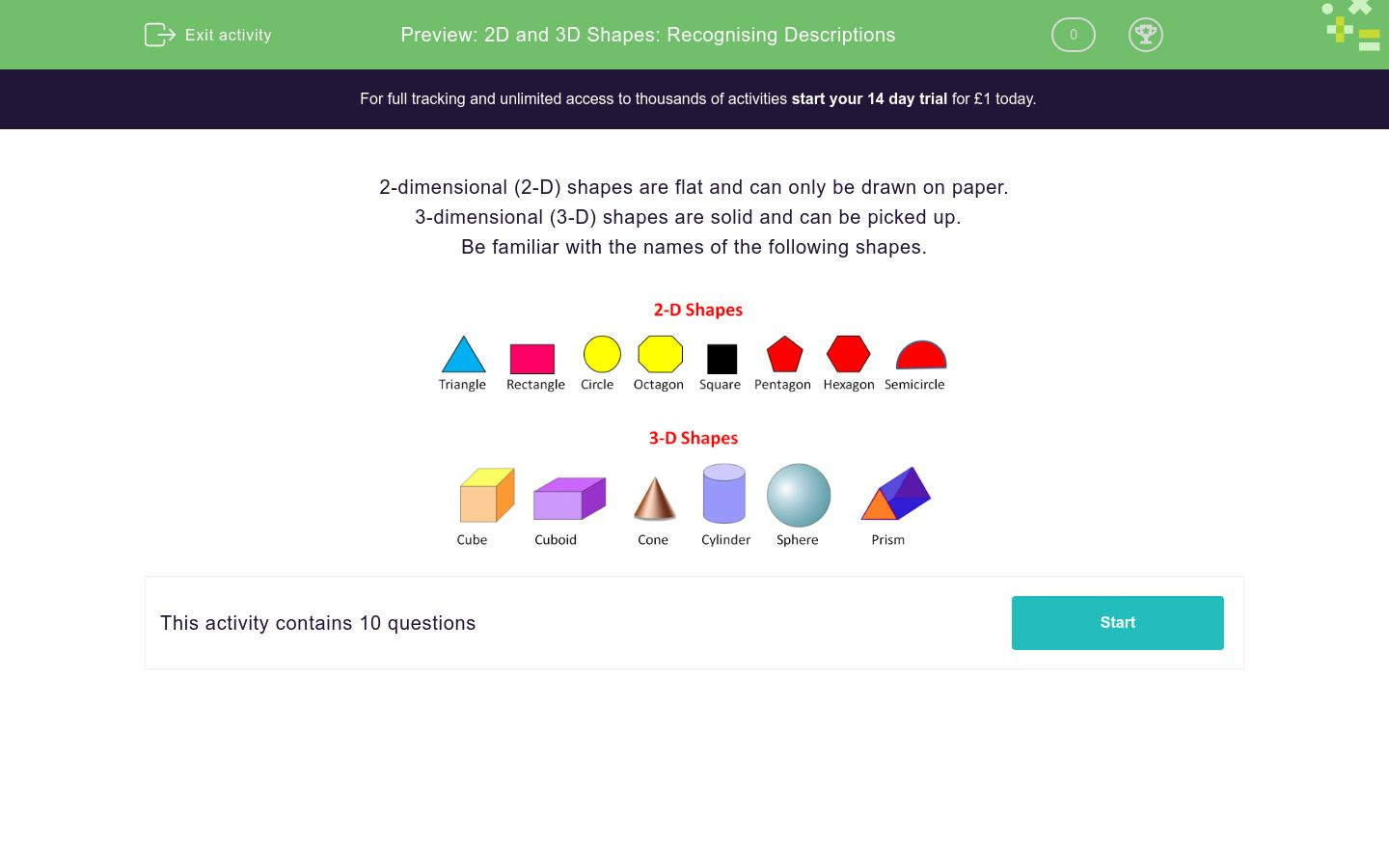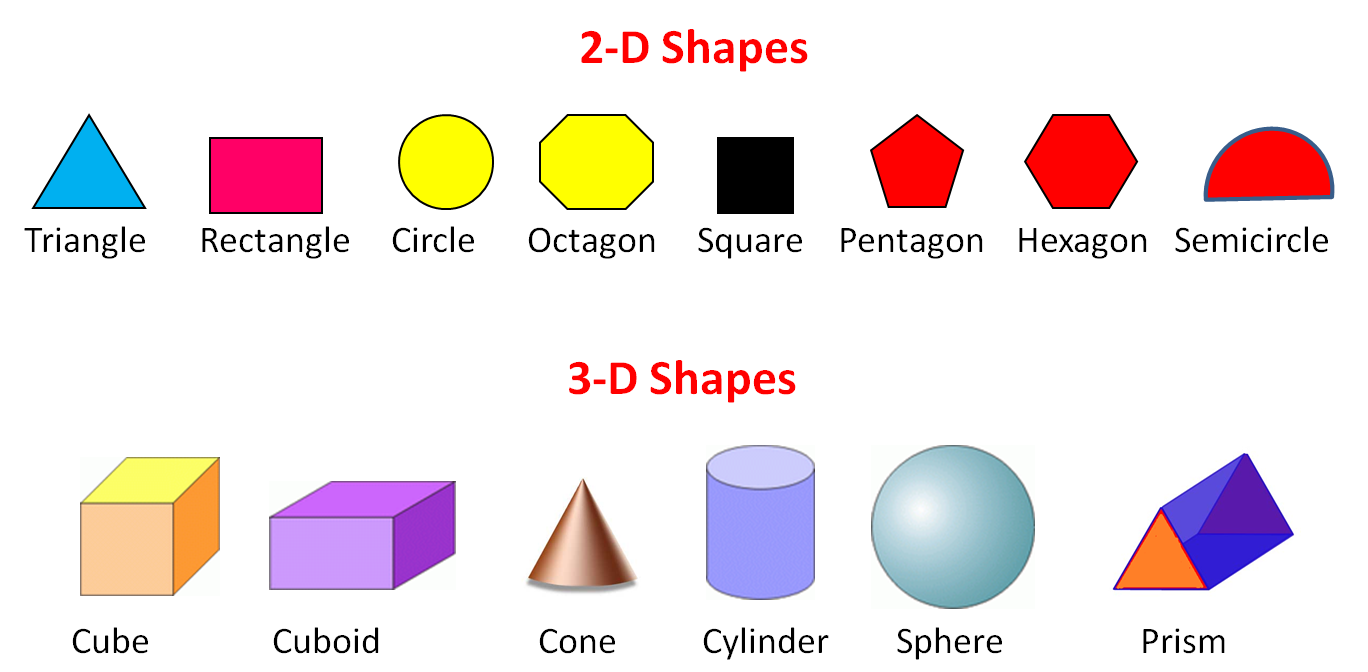# 2D and 3D Shapes: Recognising Descriptions

In this worksheet, students name the 2D and 3D shapes from the given clues.Key stage:  KS 2

Curriculum topic:   Geometry: Properties of Shapes

Curriculum subtopic:   Compare Shape Properties

Difficulty level:### QUESTION 1 of 10

2-dimensional (2-D) shapes are flat and can only be drawn on paper.

3-dimensional (3-D) shapes are solid and can be picked up.

Be familiar with the names of the following shapes.Name the following shape:

2-d with six sides

Pentagon

Hexagon

Cube

Name the following shape:

3-d with six faces, which are not all the same

Cuboid

Hexagon

Cube

Name the following shape:

3-d with six identical faces

Pentagon

Hexagon

Cube

Name the following shape:

2-d with five sides

Pentagon

Hexagon

Cube

Name the following shape:

3-d and round

Circle

Pyramid

Sphere

Name the following shape:

2-d and round

Circle

Pyramid

Sphere

Name the following shape:

Any 2-d shape with straight sides

Rectangle

Polygon

Name the following shape:

A 2-d shape with 8 corners

Pentagon

Polygon

Octagon

Name the following shape:

A 3-d shape with 8 corners

Cuboid

Cone

Octagon

Name the following shape:

A 2-d shape which is half a circle

Hemicircle

Demicircle

Semicircle

• Question 1

Name the following shape:

2-d with six sides

Hexagon
EDDIE SAYS
2-d with six sides - hexagon
• Question 2

Name the following shape:

3-d with six faces, which are not all the same

Cuboid
EDDIE SAYS
3-d with six faces, which are not all the same - cuboid
• Question 3

Name the following shape:

3-d with six identical faces

Cube
EDDIE SAYS
3-d with six identical faces - cube
• Question 4

Name the following shape:

2-d with five sides

Pentagon
EDDIE SAYS
2-d with five sides - pentagon
• Question 5

Name the following shape:

3-d and round

Sphere
EDDIE SAYS
3-d and round - sphere
• Question 6

Name the following shape:

2-d and round

Circle
EDDIE SAYS
2-d and round - circle
• Question 7

Name the following shape:

Any 2-d shape with straight sides

Polygon
EDDIE SAYS
Any 2-d shape with straight sides - polygon
• Question 8

Name the following shape:

A 2-d shape with 8 corners

Octagon
EDDIE SAYS
A 2-d shape with 8 corners - octagon
• Question 9

Name the following shape:

A 3-d shape with 8 corners

Cuboid
EDDIE SAYS
A 3-d shape with 8 corners - cuboid
• Question 10

Name the following shape:

A 2-d shape which is half a circle

Semicircle
EDDIE SAYS
A 2-d shape which is half a circle - semicircle
---- OR ----

Sign up for a £1 trial so you can track and measure your child's progress on this activity.

### What is EdPlace?

We're your National Curriculum aligned online education content provider helping each child succeed in English, maths and science from year 1 to GCSE. With an EdPlace account you’ll be able to track and measure progress, helping each child achieve their best. We build confidence and attainment by personalising each child’s learning at a level that suits them.

Get started